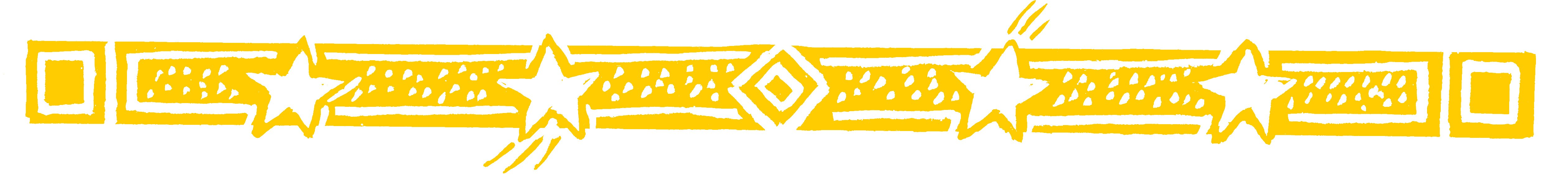Why not check your sums by this fascinating method using digit sums.

• Suppose you want to check that the simple

4  3
3  2  +
___
7  5

We find the digit sums of 43, 32 and 75 and check
that the first two digit sums add up to the third digit sum.

4  3           7
3  2  +       5 +
___           _
7  5           3

The digit sums are shown on the right and
7 + 5 = 3 is correct in digit sums because
7 + 5 = 12 = 3 (1 + 2 = 3)

This indicates that the answer is correct.

All sums, even the most complex, can be checked in this way.

Question Comment !
 Tutorial Title Question Title No Questions 0 Questions Asked 0 Skipped Questions 0 Correctly Answered 0 Wrongly Answered 0 Total Question Attempts 0 Current Question Attempts 0

Skip Question

Reset Test

Halt Test

 No Questions 0 Questions Asked 0 Skipped Questions 0 Correctly Answered 0 Wrongly Answered 0 Total Question Attempts 0

Time Taken

CloseIn Your Maths Lesson

It is worth noting (in case you want to check
subtraction sums using the nine-point circle) that in
digit sums  7-5=2, 6-6=0 and so on, but 3-4=8.
You can see this on the circle by starting at 3 and
going 4 jumps backwards round the circle.
Or, alternatively you can add 9 to the 3 so that 3-4
becomes 12-4, which is 8.and perfection of
Mathematics are
intimately
connected with the
prosperity of the
state
NAPOLEON